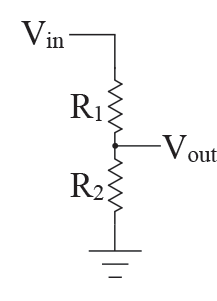# Voltage Divider CalculatorVolts

Volts

To calculate the Input Voltage enter Resistor R1, Resistor R2 and Output Voltage and then click Calculate Values.

To calculate the Output Voltage enter Input Voltage, Resistor R1 and Resistor R2 and then click Calculate Values.

To calculate the value for Resistor R1 enter Input Voltage, Resistor R2 and Output Voltage and then click Calculate Values.

To calculate the value for Resistor R2 enter Input Voltage, Resistor R1 and Output Voltage and then click Calculate Values.